### Home > AC > Chapter 8 > Lesson 8.2.1 > Problem8-49

8-49.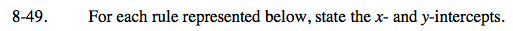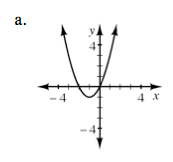Where does the relation intersect the y-axis? the x-axis? Does it intersect with the same axis multiple times?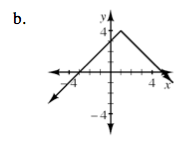y-intercept: (0, 3)
x-intercepts: (−3, 0) (5, 0)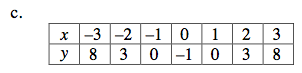Look for the zeroes in the table.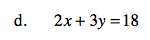To find the y-intercept, set x = 0.
To find the x-intercept, set y = 0.